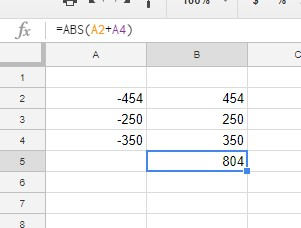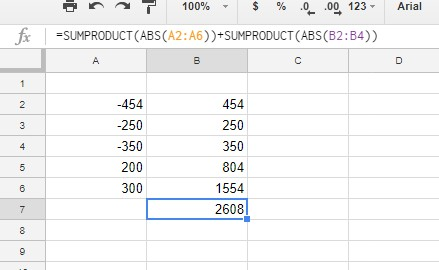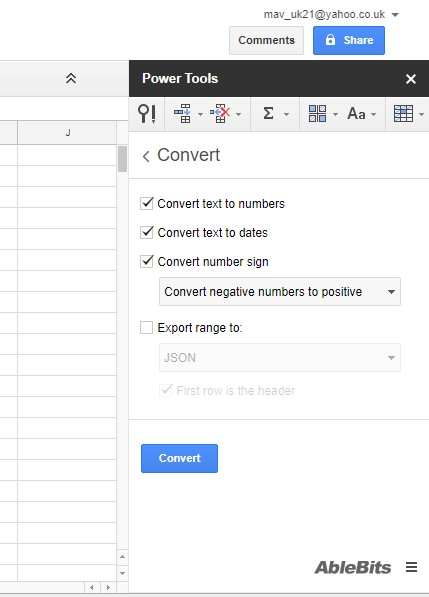# How To Get Absolute Value in Google Sheets

One mathematical concept that many people have difficulty in understanding is the notion of “absolute value”. Fortunately, an absolute value is actually a pretty simple concept. Absolute value is the distance between the number and zero. Since distance can’t be negative, absolute value is always a positive number – so the absolute value of 5 is 5 and the absolute value of -5 is also 5. That is, as a practical matter, “absolute value” means removing the negative sign in front of the number.What if you want to use absolute values in your Google Sheets spreadsheets? Well, then you’re in luck because there are actually three simple ways to accomplish this task. In this article, I will give you a brief tutorial on all three methods of obtaining absolute value in Google Sheets.

### The ABS Function in Google Sheets

ABS is a function in Google Sheets that returns the absolute value of a number.

You can always just manually convert negative numbers to positive ones, and that would work fine if you were just trying to get an absolute value for one or two cells. However, imagine having a large spreadsheet with a table column that included 350 negative numbers.

Then you would need to manually edit 350 cells in the table, which might take a while! Luckily, Google Sheets includes an ABS function so that you can quickly get absolute values for minus numbers without editing their cells. It’s a basic function that you can enter with this syntax: `=ABS(value)`. The ABS value can either be a cell reference or a number.

For some examples, open a blank spreadsheet in Google Sheets. Then enter the values ‘-454,’ ‘-250’ and -‘350‘ in the cells A2:A4 as shown in the snapshot directly below. Now you can convert that dummy data to absolute values.Select cell B2 and enter the function `=ABS(A2)` in the fx bar. B2 will return the absolute value 454 when you press Enter. That’s the absolute value of -454 entered in A2.

Copy the function into the other cells with the fill handle. Select B2, left-click the cell’s bottom right corner and drag the cursor over B3 and B4.

Then release the left mouse button to copy the ABS function into those cells as shown in the screenshot below. This Tech Junkie guide provides further details for utilizing the fill handle in Google Sheets.ABS also calculates absolute values for the results of calculations. For example, select B5, enter `=ABS(>A2A4)` in the function bar and press Return. B5 will return the absolute value of 804. The SUM function would return -804, but as an absolute value, the result is 804.ABS doesn’t add a range of numbers within a single cell reference. Furthermore, a cell range might include a mixture of positive and negative numbers. As such, a SUMPRODUCT and ABS formula is perhaps the best way to add a series of numbers together for an absolute value result.

Before you add the SUMPRODUCT formula to your spreadsheet, enter ‘200’ in cell A5 and ‘300’ in A6. Then enter the formula `SUMPRODUCT≈(ABS A2:A6))` in cell B6, and press Return. B6 now adds the cell range A2:A6 and returns an absolute value of 1,554.You can also expand the formula so that it adds two, or more, cell ranges. Select cell B7 in your Sheets spreadsheet, and input the function `=SUMPRODUCT(ABS(A2:>A6))SUMPRODUCT(ABS(B2:B4))` in the function bar. The formula will add the numbers in the ranges A2:A6 and B2:B4 then return an absolute value total which in this case is 2,608.### Convert the Negative numbers to positive values

An option that converts negative numbers to positive alternatives would be a handy addition to Sheets’ toolbar. With such an option you could quickly remove the minus (-) from lots of cells to add absolute values to a spreadsheet. Power Tools is a Sheets add-on with plenty of tools, and it also includes an option that converts number signs. To use this add-on, first, add Power Tools to Google Sheets, then follow these instructions to convert negative numbers to positive numbers:

2. Select Power Tools
3. Select Convert from the pull-down menu to open Power Tools as in the screenshot below. That opens the add-on’s Convert options
4. Click the Convert number sign checkbox
5. Then select Convert negative numbers to positive from the drop-down menu
6. Next, select the cell range A2:A4 on your Sheets spreadsheet with the cursor
7. Finally, click the Convert button on the add-on’s sidebarThis process removes the negative signs from the cells A2:A4 as shown in the screenshot below. Thus, those cells now include absolute values rather than negative values. With this conversion option, you can quickly get the absolute values for a large range of cells without entering any ABS function in an adjacent column. The Power Tools Add-on has become an essential tool for Google Sheets power users.So you can get absolute values in Sheets with either the ABS function or Power Tools without manually editing cells. If you use Excel, you might find How to get Absolute Value in Excel to be a useful TechJunkie tutorial.

Do you have any Google Sheets tips and tricks that you find useful? Please comment below.

Disclaimer: Some pages on this site may include an affiliate link. This does not effect our editorial in any way.

Todays Highlights